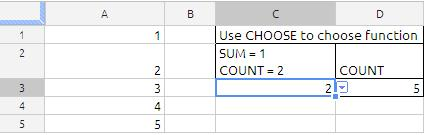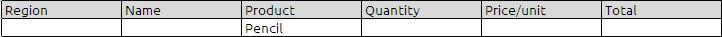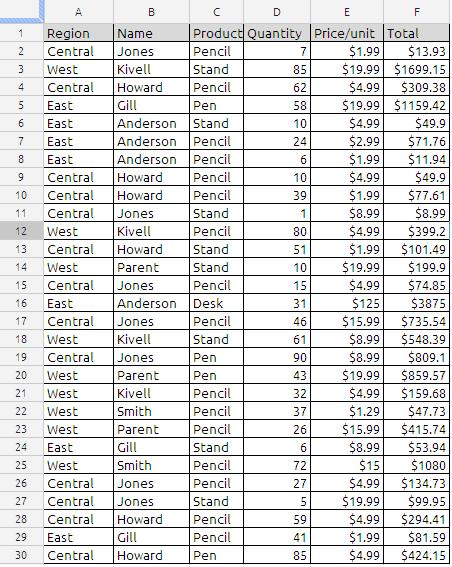## CHOOSE

The syntax of the function Choose is as follow
CHOOSE(index, value1, … value30)

Uses an index to return a value from a list of up to 30 values. Index is a reference or number between 1 and 30 indicating which value is to be taken from the list. Value1, … value30 is the list of values entered as a reference to a cell or as individual values.

CHOOSE can return a value or a range from a list of 1 to 30 arguments.

When the “lookup_value” / index_number is whole number between 1 and 30, CHOOSE can be a good lookup tool

If index_num is 1, then value1 is used …
If index_num is 2, then value2 is used

value can be a number, or a formula/function, “text” (in quotes), a range, cell references, or defined names

To find next friday from any given day we can use Choose function
Suppose A1 hold the 1/1/2012 (mm/dd/yyyy)
the following formula will give the next friday

=CHOOSE(WEEKDAY(A1),A1+5,A1+4,A1+3,A1+2,A1+1,A1,A1+6,A1+7)

if we have data down as belowThe formula in cell D2 is
=CHOOSE(C3,”SUM”,”COUNT”)
if the cell C3 is 1 we will get SUM and if cell C3 is 2 we will get COUNT

The formula in cell D3 is
=CHOOSE(C3,SUM(A1:A5),COUNT(A1:A5))
In the example shown above the cell C3 is 2 so we will get the count of cells from A1 TO  A5
if the cell value in C3 is 1 we will get the SUM of cells from A1 to A5

## COUNTIF

Returns the number of elements that meet certain criteria within a cell range. Range is the range to which the criteria are to be applied.

COUNTIF(range, criteria)

range:  The collection containing the values to be tested. Range  is a collection that can contain any value type.

criteria:  An expression that results in a logical TRUE or FALSE. condition is an expression that can contain anything as long as the result from comparing condition to a value in range can be expressed as a Boolean value of TRUE or FALSE.

Usage Notes
Each range value is compared to criteria. If the value meets the conditional test, it is included in the count.

You can use the wildcard characters, question mark (?) and asterisk (*), in criteria. A question mark matches any single character; an asterisk matches any sequence of characters. If you want to find an actual question mark or asterisk, type a tilde (~) before the character.

Examples:
In the sample data if we want to count for the criteria “Pencil “

the formula will be
=COUNTIF(C2:C30,”Pencil”) will return value 16

the same results can be achieved using other worksheet formulas ex:
Using Sumproduct
=ArrayFormula(SUMPRODUCT((C2:C30=”Pencil”)))

Using Sum
=ArrayFormula(SUM((C2:C30=”Pencil”)))

Using DCOUNTA
=DCOUNTA(A1:F30,”Product”,K2:P3)
where K2:P3 iswith wildcard (*,?)
if we want to count the word Pen and Pencil in the countif
=COUNTIF(C2:C30,”Pen*”) will return 20
Count will include the both Pen and Pencil

To Count the unique values in the column E which contain only numbers the formula will be
=ArrayFormula(sum(1/countif(E2:E30,E2:E30))) will return with answer of 9

To Count the unique values in the column c which contain text the formula will be

=ArrayFormula(SUM((C2:C30<>””)/COUNTIF(C2:C30,C2:C30&””))) will return with value 4
this can be cross checked with =unique(c3:c30)

To count the number of cells that contain a negative number:

=COUNTIF(data,”<0″)

To count the number of cells that contain the word “yes” (not case sensitive):

=COUNTIF(range,”yes”)

To count the number of cells that contain any text:

=COUNTIF(range,”*”)

To count the number of cells that contain either “yes” or “no” (not case-sensitive):

=COUNTIF(range,”yes”)+COUNTIF(range,”no”)

To count the number of cells that contain a value between 1 and 10:

=COUNTIF(range,”>=1″)-COUNTIF(range,”>10″)

Sample data## SUMIF FUNCTION

The SUMIF function returns the sum of a collection of numbers, including only numbers that satisfy a specified condition.

SUMIF(range, criteria, sum_range)

range:  The collection containing the values to be tested. range-values is a collection containing any value type.

criteria:  An expression that results in a logical TRUE or FALSE. criteria is an expression that can contain anything as long as the result from comparing criteria to a value in range can be expressed as a Boolean value of TRUE or FALSE.

sum-range:  An optional collection containing the numbers to be summed. Sumrange is a collection containing number, date/time, or duration values. It should have the same dimensions as range.

Notes:
Although range can contain any type of value, it should usually contain values all of the same type. If the sum_range is partially entered then the range will be taken as sum-range

Important 1:
Criteria has to be in quotes unless you put criteria  in  a cell  and refer to it with a cell reference.

Important 2: Criteria for greater than 10 could be :
1)”>10”
2)”>”&A1 where 10 was in cell A1
3) A1 where >10 was in cell A1

Example:
In the sample data if we want to sum total value for the criteria “Pensil “

Formula will be
=sumif(C2:C30,”Pencil”,F2:F30)
To make more generalized formula we can also write as
=sumif(C2:C,”Pencil”,F2:F)
or
=sumif(C2:C30,”Pencil”,F2)
In the third argument (sum-range) if we ignore the whole range still function will works and the function will treat sum-range as range values

other way for achieving the same results for the above stated criteria
Using Sum Function
traditional way of writing with if function
=ArrayFormula(sum(if(C2:C30=”Pencil”,(F2:F30))))
or
=ArrayFormula(SUM((C2:C30=”Pencil”)*(F2:F30)))

Using Sumproduct Function
=ArrayFormula(SUMPRODUCT((C2:C30=”Pencil”)*(F2:F30)))

Using Filter function
=sum(filter(F2:F,C2:C=”Pencil”))

Using Query function
=sum(query(A:F,”select F where C = ‘Pencil'”)

Using DSUM Function
=dsum(A1:F30,”Total”,K2:P3)
where K2:P3 will as below## Time Math in google docs

Now, lets look at the values and formulas in each column.  Column B is simply the Name of each employee.  Columns C, D, E, and F are the times that each employee started work, left for lunch, returned from lunch, and left work for the day.   Pay attention to the hours worked by Emma, in row 8.  Her shift extended over midnight (she started at 10PM and left at 7AM), and her lunch break also extended over midnight.  Our formulas will take this into account, and calculate the proper times.

Cell H2 contains the number of regular, non-overtime, hours per shift.  Here, the 8 indicates that a normal shift is 8 hours.  Cell K2 contains the rate at which overtime hours are paid.  The 1.5 indicates that overtime is paid at time-and-a-half.

The total hours are in column G.  This is the difference between the start time in column B and the end time in column F.  This value does not pay any attention to the time taken for lunch — we’ll do that later.  The formula in G4 is:

=(F4-C4+(F4<C4))*24

This formula subtracts the start time in C4 from the end time in F4.  Then, it compares the end time to the start time.  If F4 is less than, or earlier than, C4, the expression(F4<C4) will evaluate to True or 1.  If not, it will evaluate to False or 0. Adding this comparison result will calculate the number of hours properly in the case where the start time is later in the day than the end time.  This is exactly the situation with Emma.  Finally, the result is multiplied by 24, to get the actual number of hours, rather than a time value — e.g., 9.00 rather 9:00.  (Since times are stored as a fraction of a 24-hour day, multiplying a time by 24 will return the number of hours).

NOTE: You only need to use the +(F4<C4)part of the formula when the start and end times are just times, without a date.  If you are entering a complete date and time, then you don’t need the comparison formula, because the date part of the value will allow Excel to properly calculate the difference.

Column H contains the number of hours that were actually worked, after taking out the time taken for lunch.  The formula in H4 is:
=((F4-C4+(F4<C4))-(E4-D4+(E4<D4)))*24

This formula is very similar to the formula in G4, expect that it subtracts the time between D4 and E4, the start and end times of the lunch break.  Again, we compare the start and end times to ensure that the number of hours is calculated properly if the time period crosses midnight.  Look at Emma’s hours again — she left for lunch at 11PM and returned at 12:30 AM.   Multiplying the result by 24 gives us the actual number of hours worked, rather than a time value.

Column I contains the number of regular hours worked by each employee.  If the actual worked hours in column H is less than the Regular Hours value in H2, we want to use the actual number of worked hours.  However, if the actual worked hours in column H is greater than the Regular Hours value in H2, we only want to count H2 hours as regular time.  The formula in I4 is:

=MIN(\$H\$2,H4)

This formula simply takes the minimum of the allowed regular hours in H2 and the number of hours actually worked in H4.

Column J contains the number of overtime hours for each employee.  If the employee worked less than the allowed regular hours, the overtime hours will, of course, be zero.  However, if they worked more than the allowed regular hours, the overtime hours will be the difference between the hours actually worked and the allowed regular hours.  The formula in cell J4 is:

=MAX(0,H4-\$H\$2)

This is simply the maximum of 0 and the difference between actual hours and allowed regular hours.

Column K is just the hourly wage for each employee.  Of course, these may be different for each employee, but they are the same in our example. Column L is the amount of regular, non-overtime wages paid.  This is just the product of column I, the regular hours, and column K, the hourly wage.  Cell L4 has the formula:

=I4*K4

Column M is the amount of overtime wages paid.  This is the product of column J, the overtime hours, column K, the hourly wage, and cell K2, the rate at which overtime hours are paid. Cell M4 has the formula:

=J4*K4*\$K\$2

Finally, column N is just the total wages paid, the sum of the regular and overtime wages.  Cell N4 has the formula:
=L4+M4

## QUOTIENT

The QUOTIENT function returns the integer quotient of two numbers.

QUOTIENT(numerator, denominator)

numerator: A number to be divided by another number. numerator is a number value.

denominator: A number to divide into another number. denominator is a number value. If 0, a division by zero will result and the function will return an error.

If either, but not both, the numerator or denominator is negative, the result will be negative. If the sign of both the numerator and the denominator is the same, the result will be positive.

Only the whole part of the quotient is returned. The fractional part (or remainder) is ignored.

Examples
=QUOTIENT(5, 2) returns 2.
=QUOTIENT(5.99, 2) returns 2.
=QUOTIENT(-5, 2) returns -2.
=QUOTIENT(6, 2) returns 3.